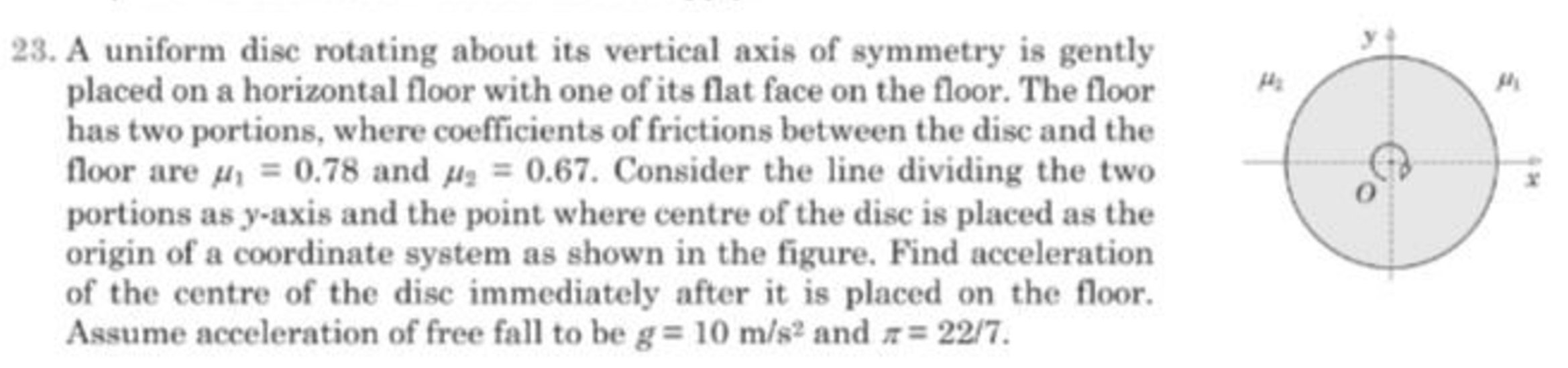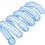# Mechanics Problem 23Position and velocity of a point on the disk:

$x = r \cos \theta \\ y = r \sin \theta \\ \dot{x} = -r \sin \theta \, \dot{\theta} \\ \dot{y} = r \cos \theta \, \dot{\theta}$

Since the friction force opposes the velocity, define a unit vector opposite the velocity.

$v = \sqrt{\dot{x}^2 + \dot{y}^2} \\ u_x = - \dot{x} / v \\ u_y = - \dot{y} / v$

Let the mass of the disk be $M$ and the radius of the disk be $R$. Derive the mass and normal force for a patch of the disk as follows:

$\rho = \frac{M}{\pi R^2} \\ dA = r \, dr \, d \theta \\ dm = \rho \, dA \\ dN = dm \, g$

If $x > 0$, the infinitesimal vector force on a patch of disk is:

$dF_x = \mu_1 \, dN \, u_x \\ dF_y = \mu_1 \, dN \, u_y$

If $x \leq 0$, the infinitesimal vector force on a patch of disk is:

$dF_x = \mu_2 \, dN \, u_x \\ dF_y = \mu_2 \, dN \, u_y$

The total force is then:

$F_x = \int_0^{2 \pi} \int_0^R dF_x \\ F_y = \int_0^{2 \pi} \int_0^R dF_y$

This could probably be done analytically, but I did it numerically instead. The answer is independent of the particular values of $M$, $R$, and $\dot{\theta}$. The initial acceleration of the center of mass is approximately $0.35$.

  1 2 3 4 5 6 7 8 9 10 11 12 13 14 15 16 17 18 19 20 21 22 23 24 25 26 27 28 29 30 31 32 33 34 35 36 37 38 39 40 41 42 43 44 45 46 47 48 49 50 51 52 53 54 55 56 57 58 59 60 61 62 63 64 65 66 67 68 69 70 71 72 73 74 75 76 77 78 79 80 81 82 83 84 85 86 87 88 89 90 91 92 93 94 95 96 97 98 99 100 101 102 103 104 105 106 107 108 109 110 111 import math import random Num = 5000 u1 = 0.78 u2 = 0.67 pi = 22.0/7.0 g = 10.0 m = 1.0 + 2.0*random.random() R = 1.0 + 2.0*random.random() A = pi*(R**2.0) rho = m/A thetad = 1.0 + 5.0*random.random() dtheta = 2.0*math.pi/Num dr = R/Num ################################### Fx = 0.0 Fy = 0.0 r = dr while r <= R: theta = 0.0 while theta <= 2.0*math.pi: x = r*math.cos(theta) y = r*math.sin(theta) xd = -r*math.sin(theta)*thetad yd = r*math.cos(theta)*thetad v = math.hypot(xd,yd) ux = -xd/v uy = -yd/v dA = r*dr*dtheta dm = rho*dA dN = dm*g if x > 0.0: dF = u1*dN else: dF = u2*dN dFx = dF*ux dFy = dF*uy Fx = Fx + dFx Fy = Fy + dFy theta = theta + dtheta r = r + dr ################################### xdd = Fx/m ydd = Fy/m a = math.hypot(xdd,ydd) print Num print "" print xdd print ydd print "" print a ################################### #>>> #5000 #3.93753857887e-13 #-0.349929953951 #0.349929953951 #>>> ================================ RESTART ================================ #>>> #5000 #3.93609652909e-13 #-0.349929953951 #0.349929953951 #>>> ================================ RESTART ================================ #>>> #10000 #5.7533637902e-13 #-0.349964988488 #0.349964988488 #>>> ================================ RESTART ================================ #>>> #10000 #5.75344675709e-13 #-0.349964988488 #0.349964988488 #>>>Note by Steven Chase
8 months, 2 weeks ago

This discussion board is a place to discuss our Daily Challenges and the math and science related to those challenges. Explanations are more than just a solution — they should explain the steps and thinking strategies that you used to obtain the solution. Comments should further the discussion of math and science.

When posting on Brilliant:

• Use the emojis to react to an explanation, whether you're congratulating a job well done , or just really confused .
• Ask specific questions about the challenge or the steps in somebody's explanation. Well-posed questions can add a lot to the discussion, but posting "I don't understand!" doesn't help anyone.
• Try to contribute something new to the discussion, whether it is an extension, generalization or other idea related to the challenge.
• Stay on topic — we're all here to learn more about math and science, not to hear about your favorite get-rich-quick scheme or current world events.

MarkdownAppears as
*italics* or _italics_ italics
**bold** or __bold__ bold
- bulleted- list
• bulleted
• list
1. numbered2. list
1. numbered
2. list
Note: you must add a full line of space before and after lists for them to show up correctly
paragraph 1paragraph 2

paragraph 1

paragraph 2

[example link](https://brilliant.org)example link
> This is a quote
This is a quote
    # I indented these lines
# 4 spaces, and now they show
# up as a code block.

print "hello world"
# I indented these lines
# 4 spaces, and now they show
# up as a code block.

print "hello world"
MathAppears as
Remember to wrap math in $$ ... $$ or $ ... $ to ensure proper formatting.
2 \times 3 $2 \times 3$
2^{34} $2^{34}$
a_{i-1} $a_{i-1}$
\frac{2}{3} $\frac{2}{3}$
\sqrt{2} $\sqrt{2}$
\sum_{i=1}^3 $\sum_{i=1}^3$
\sin \theta $\sin \theta$
\boxed{123} $\boxed{123}$

## Comments

Sort by:

Top Newest

@Talulah Riley Here is my solution for Problem 23

- 8 months, 2 weeks ago

Log in to reply

@Steven Chase Thank you so much for the solution . By the way check your last 8 hour notifications.

- 8 months, 2 weeks ago

Log in to reply

@Talulah Riley Hey; try my latest problem.

- 8 months, 2 weeks ago

Log in to reply

Tricky problem. Nice solution; I can't think of doing it by hand, however.

- 8 months, 2 weeks ago

Log in to reply

Your line number 18 is wrong. The question says that we should use the approximation $\pi = \frac{22}7$, But you took $\pi$ as $3.141592653\ldots$.

The same issue arise in line number 31.

- 8 months, 2 weeks ago

Log in to reply

Ah yes, old habits die hard

- 8 months, 2 weeks ago

Log in to reply

×

Problem Loading...

Note Loading...

Set Loading...